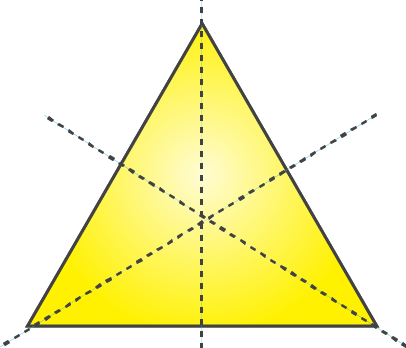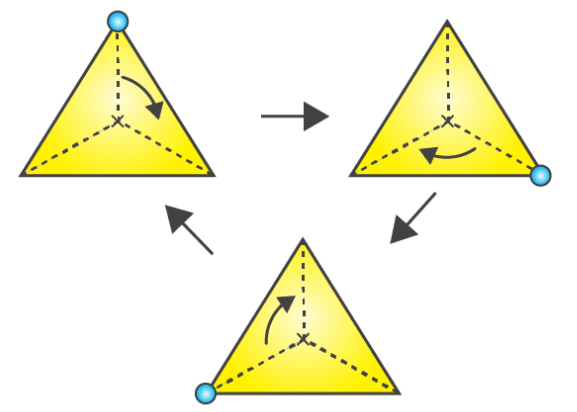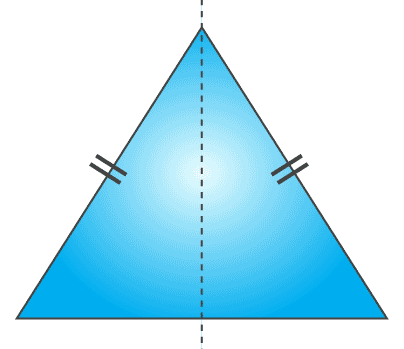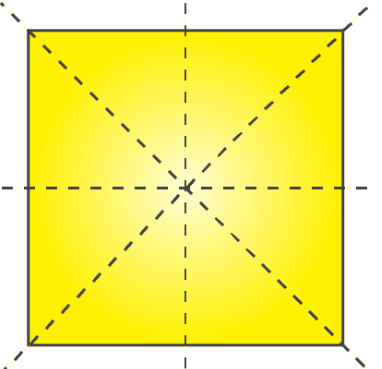## Access Answers to NCERT Solutions for Class 7 Maths Chapter 14 – Symmetry Exercise 14.3

### Access Answers to NCERT Solutions for Class 7 Maths Chapter 14 – Symmetry Exercise 14.3

1. Name any two figures that have both line symmetry and rotational symmetry.

Solution:-

Equilateral triangle and Circle.

2. Draw, wherever possible, a rough sketch of

(i) a triangle with both line and rotational symmetries of order more than 1.

Solution:-

A triangle with both line and rotational symmetries of order more than 1 is an equilateral triangle.

Line symmetryRotational symmetry(ii) a triangle with only line symmetry and no rotational symmetry of order more than 1.

Solution:-

A triangle with only line symmetry and no rotational symmetry of order more than 1 is isosceles triangle.(iii) a quadrilateral with a rotational symmetry of order more than 1 but not a line symmetry.

Solution:-

A quadrilateral with a rotational symmetry of order more than 1 but not a line symmetry is not possible to draw. Because, a quadrilateral with a line symmetry may have rotational symmetry of order one but not more than one.

(iv) a quadrilateral with line symmetry but not a rotational symmetry of order more than 1.

Solution:-

A quadrilateral with line symmetry but not a rotational symmetry of order more than 1 is rhombus.3. If a figure has two or more lines of symmetry, should it have rotational symmetry of order more than 1?

Solution:-

Yes. If a figure has two or more lines of symmetry, then it will have rotational symmetry of order more than 1.

4. Fill in the blanks:

 Shape Centre of Rotation Order of Rotation Angle of Rotation Square Rectangle Rhombus Equilateral Triangle Regular Hexagon Circle Semi-circle

Solution:-

 Shape Centre of Rotation Order of Rotation Angle of Rotation Square Intersecting point of diagonals 4 90o Rectangle Intersecting point of diagonals 2 180o Rhombus Intersecting point of diagonals 2 180o Equilateral Triangle Intersecting point of medians 3 120o Regular Hexagon Intersecting point of diagonals 6 60o Circle Centre Infinite Every angle Semi-circle Mid-point of diameter 1 360o

5. Name the quadrilaterals which have both line and rotational symmetry of order more than 1.

Solution:-

The quadrilateral which have both line and rotational symmetry of order more than 1 is square.

Line symmetry:Rotational symmetry:6. After rotating by 60° about a centre, a figure looks exactly the same as its original position. At what other angles will this happen for the figure?

Solution:-

The other angles are, 120°, 180°, 240°, 300°, 360°

So, the figure is said to have rotational symmetry about same angle as the first one. Hence, the figure will look exactly the same when rotated by 60° from the last position.

7. Can we have a rotational symmetry of order more than 1 whose angle of rotation is

(i) 45°?

Solution:-

Yes. We can have a rotational symmetry of order more than 1 whose angle of rotation is 45o.

(ii) 17°?

Solution:-

No. We cannot have a rotational symmetry of order more than 1 whose angle of rotation is 17o.